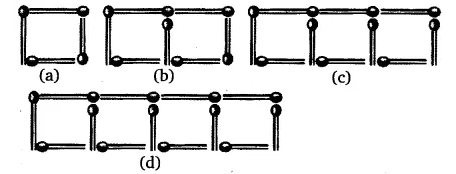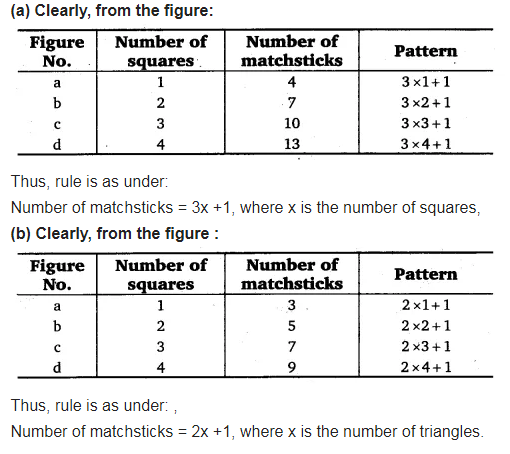# (a) Look at the following matchstick pattern of squares (see figure). The squares are not separate

(a) Look at the following matchstick pattern of squares (see figure). The squares are not separate. Two neighbouring squares have a common matchstick. Observe the pattern and find the rule that gives the number of matchsticks in terms of the number of squares.(b) Figure gives a matchstick pattern of triangles. As in Exercise 11(a) above, find the general rule that gives the number of matchsticks in terms of the number of triangles.